# Civil Engineering - UPSC Civil Service Exam Questions

16.

A close-coiled helical spring has 100 mm mean diameter and is mada of 20 turns to 10 mm diameter steel wire. The spring carries an axial load of 100 N Modulus of rigidity is 84 GPa. The shearing stress developed in the spring in N/mm2 is

 A. 120/π B. 160/π C. 100/π D. 80/π

Explanation:

No answer description available for this question. Let us discuss.

17.

Consider the following statements pertaining to plane table survey :
1. Two-point problem is solved by mechanical method.
2. Three-point problem is solved by Bessel's method.
3. In two point problem, auxiliary station is required.
Of these statements :

 A. 1 and 2 are correct B. 1 and 3 arc correct C. 2 and 3 are correct D. 1, 2 and 3 are correct

Explanation:

No answer description available for this question. Let us discuss.

18.

If a member is subjected to tensile stress of px, compressive stress of py and tensile stress of pz along the x, y and z directions respectively, then the resultant strain 'ex' along the 'x' direction would be (E is Young's modulus of elasticity, μ is Poisson's ratio)

 A.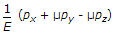B.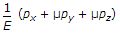C.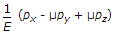D.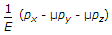Explanation:

No answer description available for this question. Let us discuss.

19.

A cantilever is subjected to a uniformly distributed load W over its whole length 'L' and a concentrated upward force W at its free end. The deflection of the free end is

 A. zero B.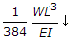C.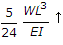D.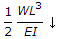Explanation:

No answer description available for this question. Let us discuss.

20.

The local sidereal time (LST) is always

 A. ahead of the local mean time (LMT) by about 2 hours per month B. behind LMT by about 2 hours per month C. ahead of LMT by about 4 hours per month D. equal to LMT